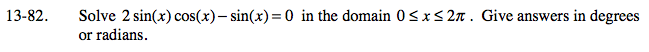### Home > A2C > Chapter 13 > Lesson 13.1.4 > Problem13-82

13-82.

Solve 2 sin(x) cos(x) −sin(x) = 0 in the domain 0 ≤ x 2π . Give answers in degrees or radians. Homework Help ✎sin(x) is a common factor. Factor and use the Zero Product Property and the unit circle to solve.

$0, \frac{\pi}{3},\,(?), (?)$